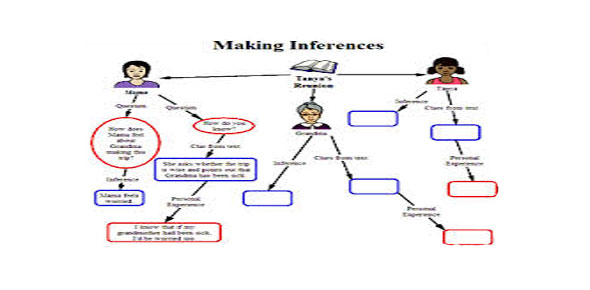# Quiz On Fuzzy Inference Diagram/Sugeno-type Fuzzy Inference

8 Questions | Total Attempts: 105SettingsYOU HAVE 10 MINUTES TO ANSWER ALL THE QUESTIONS. ENJOY THE QUIZ AND GOOD LUCK.

• 1.
In What Directions Do The Inputs Tend To Go?
• A.

Downwards

• B.

Across

• C.

Upwards

• D.

None of the above

• 2.
In Which Direction Do The Rules Tend To Go?
• A.

Downwards

• B.

Across

• C.

Upwards

• D.

None of the above

• 3.
In Which Direction Do The Outputs Tend To Go?
• A.

Downwards

• B.

Across

• C.

Upwards

• D.

None of the above

• 4.
What Is The Output For The Sugeno-Type?
• A.

Either one or zero

• B.

Value between 1 and 100

• C.

Value between 100 and 200

• D.

Either linear or constant

• 5.
What is a typical rule in Sugeno-Type?
• A.

If input 1 = x and input 2 = y, the output is z=ay+bx+c

• B.

If input 1 = x and input 2 = y, the output is z=ax+by+c

• C.

If input 1 = x and input 2 = y, the output is z=ab+xy+c

• D.

None of the above

• 6.
What Is An Advantages Of Sugeno-Type Method?
• A.

Only deals with whole numbers

• B.

Reduces the output value

• C.

Reduces the input value

• D.

It is computationally efficient

• 7.
What Is Another Advantage Of Sugeno-Type Method?
• A.

More than one value can be correct

• B.

Can be helpful with scientifical experiments

• C.

Is is well suited for mathematical analysis

• D.

None of the above

• 8.
What Is An Advantages Of Mamdani-Type Method?
• A.

It is Intaitive

• B.

It is well suited for mathmatical analysis

• C.

It is computationally efficient

• D.

None of the above

Related TopicsBack to top